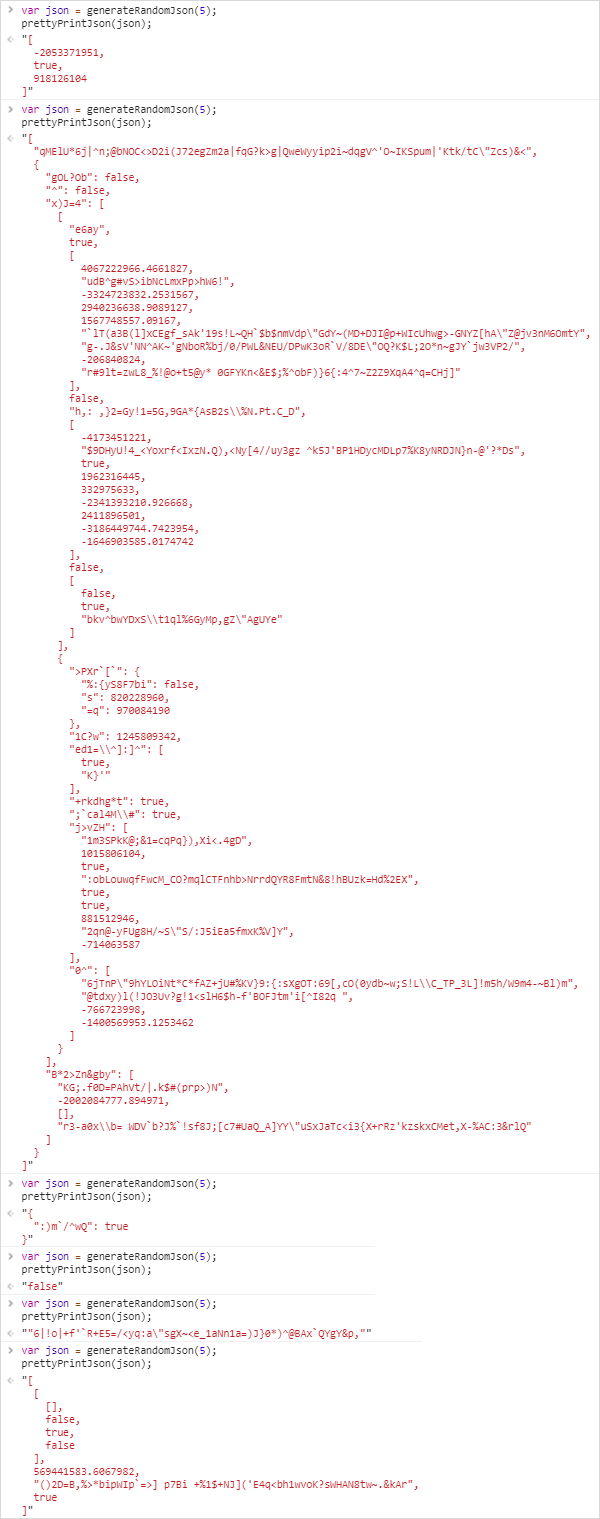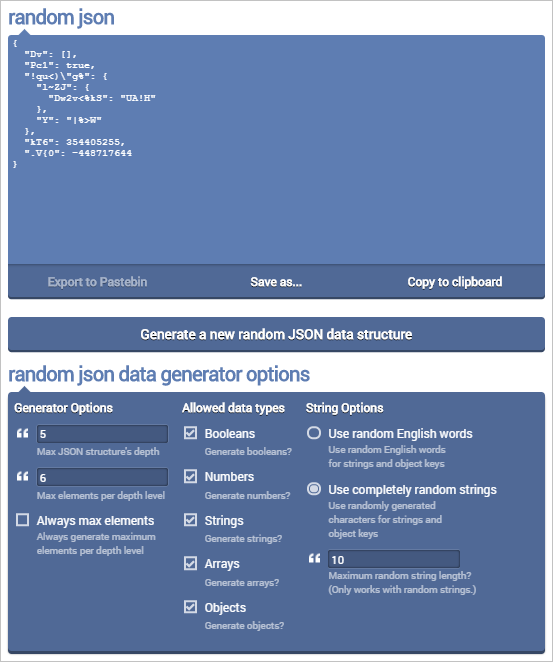Yesterday I wrote a random JSON data structure generator for my next Online Random Tools. Here’s a quick article about what my thought process was when I was writing it.

1. What’s a name for this function? `generateRandomJson` sounds good. Let’s put it in code:

```function generateRandomJson () {
```

2. So what is a structure? It’s one of number, string, boolean, array or object. So let’s put it in code:

```var choices = ["number", "string", "boolean", "array", "object"];
var choice = chooseOne(choices);

function chooseOne(choices) {
return choices[parseInt(Math.random()*choices.length)];
}
```

. What is a number? It’s an integer or a floating point. Negative or positive. Let’s put that in code:

```if (choice == "number") {
return generateRandomNumber();
}

function generateRandomNumber () {
var maxNum = 2**32;
var number = Math.random()*maxNum;
var isInteger = chooseOne([true,false]);
var isNegative = chooseOne([true,false]);

if (isInteger) number = parseInt(number);
if (isNegative) number = -number;

return number;
}
```

4. What is a string? It’s zero or more characters. Let’s put it in code:

```if (choice == "string") {
return generateRandomString();
}

function generateRandomString () {
var alphabet = " !"#\$%&'()*+,-./0123456789:;<=>?@ABCDEFGHIJKLMNOPQRSTUVWXYZ[\]^_`abcdefghijklmnopqrstuvwxyz{|}~";

var maxLength = 100;
var length = Math.random()*maxLength;
var string = "";
for (var i = 0; i < length; i++) {
string += chooseOne(alphabet);
}

return string;
}
```

5. What is a boolean? It’s true or false. Let’s put it in code:

```if (choice == "boolean") {
return generateRandomBoolean();
}

function generateRandomBoolean () {
return chooseOne([true,false]);
}
```

6. What is an array? It’s zero or more of random JSON data . Let’s put that in code:

```if (choice == "array") {
return generateRandomArray();
}

function generateRandomArray () {
var maxArrayLength = 10;
var length = parseInt(Math.random()*maxArrayLength);

var array = [];
for (var i = 0; i < length; i++) {
array[i] = generateRandomJson();
}

return array;
}
```

7. What is an object? It’s zero or more random string keys with random JSON data structures as values. Let’s put that in code:

```if (choice == "object") {
return generateRandomObject();
}

function generateRandomObject () {
var maxObjectKeys = 10;
var keyCount = parseInt(Math.random()*maxObjectKeys);

var object = {};
for (var i = 0; i < keyCount; i++) {
var key = generateRandomKeyName();
object[key] = generateRandomJson();
}

return object;
}

function generateRandomKeyName () {
var maxKeyLength = 10;
var keyLength = 1 + parseInt(Math.random()*maxKeyLength);
var randomString = generateRandomString();
return randomString.substr(0,keyLength)
}
```

8. And we’re done. We’ve covered all the cases and can close the `generateRandomJson` function:

9. Now let’s try it and pretty print it:

```var json = generateRandomJson();
console.log(prettyPrintJson(json));

function prettyPrintJson (json) {
return JSON.stringify(json, null, 2);
}
```

This function will sometimes cause a stack overflow because we haven’t limited the depth of the JSON data structure. We can easily do that by passing `maxDepth` as an argument and avoiding generating nested JSON substructures when it’s 0. There are many more improvements that can be made, such as passing options for max string length, max number to , max number of elements per depth level, etc. I’ve implemented all these in Random JSON Generator online tool that you can try.

```function generateRandomJson (maxDepth) {
var choices = ["number", "string", "boolean", "array", "object"];
if (maxDepth == 0) {
choices = ["number", "string", "boolean"];
}

var choice = chooseOne(choices);

function chooseOne(choices) {
return choices[parseInt(Math.random()*choices.length)];
}

if (choice == "number") {
return generateRandomNumber();
}
if (choice == "string") {
return generateRandomString();
}
if (choice == "boolean") {
return generateRandomBoolean();
}
if (choice == "array") {
return generateRandomArray();
}
if (choice == "object") {
return generateRandomObject();
}

function generateRandomNumber () {
var maxNum = 2**32;
var number = Math.random()*maxNum;
var isInteger = chooseOne([true,false]);
var isNegative = chooseOne([true,false]);

if (isInteger) number = parseInt(number);
if (isNegative) number = -number;

return number;
}

function generateRandomString () {
var alphabet = " !"#\$%&'()*+,-./0123456789:;<=>?@ABCDEFGHIJKLMNOPQRSTUVWXYZ[\]^_`abcdefghijklmnopqrstuvwxyz{|}~";

var maxLength = 100;
var length = Math.random()*maxLength;
var string = "";
for (var i = 0; i < length; i++) {
string += chooseOne(alphabet);
}

return string;
}

function generateRandomBoolean () {
return chooseOne([true,false]);
}

function generateRandomArray () {
var maxArrayLength = 10;
var length = parseInt(Math.random()*maxArrayLength);

var array = [];
for (var i = 0; i < length; i++) {
array[i] = generateRandomJson(maxDepth-1);
}

return array;
}

function generateRandomObject () {
var maxObjectKeys = 10;
var keyCount = parseInt(Math.random()*maxObjectKeys);

var object = {};
for (var i = 0; i < keyCount; i++) {
var key = generateRandomKeyName();
object[key] = generateRandomJson(maxDepth-1);
}

return object;
}

function generateRandomKeyName () {
var maxKeyLength = 10;
var keyLength = 1 + parseInt(Math.random()*maxKeyLength);
var randomString = generateRandomString();
return randomString.substr(0,keyLength)
}
}
```

Now let’s generate a random JSON data structure with maxDepth of 5:

```var json = generateRandomJson(5);
prettyPrintJson(json);
```

Success!I also made a nice UI for this tool. You can with it here:Clear thoughts lead to clear code. See you next time!